## The Parabola

### Learning Outcomes

• Graph parabolas with vertices at the origin.
• Write equations of parabolas in standard form.
• Graph parabolas with vertices not at the origin.
• Solve applied problems involving parabolas.

Did you know that the Olympic torch is lit several months before the start of the games? The ceremonial method for lighting the flame is the same as in ancient times. The ceremony takes place at the Temple of Hera in Olympia, Greece, and is rooted in Greek mythology, paying tribute to Prometheus, who stole fire from Zeus to give to all humans. One of eleven acting priestesses places the torch at the focus of a parabolic mirror, which focuses light rays from the sun to ignite the flame.The Olympic torch concludes its journey around the world when it is used to light the Olympic cauldron during the opening ceremony. (credit: Ken Hackman, U.S. Air Force)

Parabolic mirrors (or reflectors) are able to capture energy and focus it to a single point. The advantages of this property are evidenced by the vast list of parabolic objects we use every day: satellite dishes, suspension bridges, telescopes, microphones, spotlights, and car headlights, to name a few. Parabolic reflectors are also used in alternative energy devices, such as solar cookers and water heaters, because they are inexpensive to manufacture and need little maintenance. In this section we will explore the parabola and its uses, including low-cost, energy-efficient solar designs.

## Parabolas with Vertices at the Origin

In The Ellipse we saw that an ellipse is formed when a plane cuts through a right circular cone. If the plane is parallel to the edge of the cone, an unbounded curve is formed. This curve is a parabola.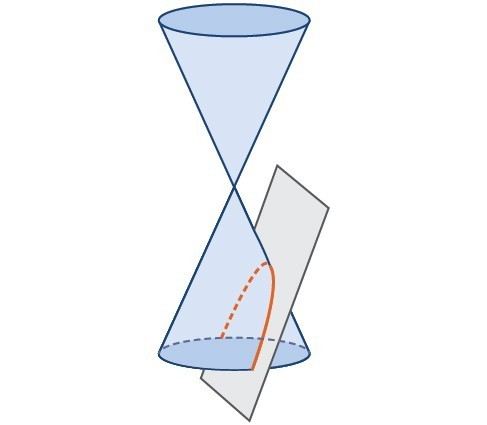Parabola

Like the ellipse and hyperbola, the parabola can also be defined by a set of points in the coordinate plane. A parabola is the set of all points $\left(x,y\right)$ in a plane that are the same distance from a fixed line, called the directrix, and a fixed point (the focus) not on the directrix.

We previously learned about a parabola’s vertex and axis of symmetry. Now we extend the discussion to include other key features of the parabola. Notice that the axis of symmetry passes through the focus and vertex and is perpendicular to the directrix. The vertex is the midpoint between the directrix and the focus.

The line segment that passes through the focus and is parallel to the directrix is called the latus rectum, also called the focal diameter. The endpoints of the focal diameter lie on the curve. By definition, the distance $d$ from the focus to any point $P$ on the parabola is equal to the distance from $P$ to the directrix.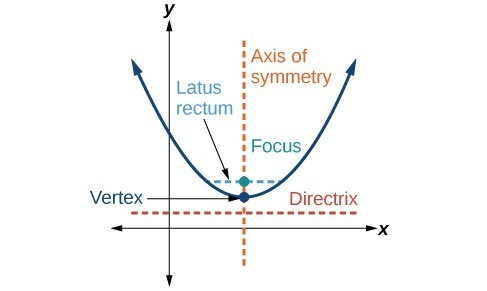Key features of the parabola

To work with parabolas in the coordinate plane, we consider two cases: those with a vertex at the origin and those with a vertex at a point other than the origin. We begin with the former.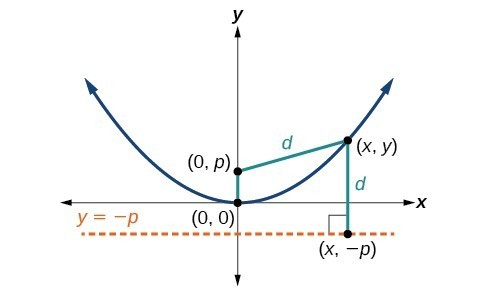Let $\left(x,y\right)$ be a point on the parabola with vertex $\left(0,0\right)$, focus $\left(0,p\right)$, and directrix $y= -p$ as shown in Figure 4. The distance $d$ from point $\left(x,y\right)$ to point $\left(x,-p\right)$ on the directrix is the difference of the y-values: $d=y+p$. The distance from the focus $\left(0,p\right)$ to the point $\left(x,y\right)$ is also equal to $d$ and can be expressed using the distance formula.

\begin{align}d&=\sqrt{{\left(x - 0\right)}^{2}+{\left(y-p\right)}^{2}} \\ &=\sqrt{{x}^{2}+{\left(y-p\right)}^{2}} \end{align}

Set the two expressions for $d$ equal to each other and solve for $y$ to derive the equation of the parabola. We do this because the distance from $\left(x,y\right)$ to $\left(0,p\right)$ equals the distance from $\left(x,y\right)$ to $\left(x, -p\right)$.

$\sqrt{{x}^{2}+{\left(y-p\right)}^{2}}=y+p$

We then square both sides of the equation, expand the squared terms, and simplify by combining like terms.

$\begin{gathered}{x}^{2}+{\left(y-p\right)}^{2}={\left(y+p\right)}^{2} \\ {x}^{2}+{y}^{2}-2py+{p}^{2}={y}^{2}+2py+{p}^{2}\\ {x}^{2}-2py=2py \\ {x}^{2}=4py\end{gathered}$

The equations of parabolas with vertex $\left(0,0\right)$ are ${y}^{2}=4px$ when the x-axis is the axis of symmetry and ${x}^{2}=4py$ when the y-axis is the axis of symmetry. These standard forms are given below, along with their general graphs and key features.

### A General Note: Standard Forms of Parabolas with Vertex (0, 0)

The table below summarizes the standard features of parabolas with a vertex at the origin.

 Axis of Symmetry Equation Focus Directrix Endpoints of Focal Diameter x-axis ${y}^{2}=4px$ $\left(p,\text{ }0\right)$ $x=-p$ $\left(p,\text{ }\pm 2p\right)$ y-axis ${x}^{2}=4py$ $\left(0,\text{ }p\right)$ $y=-p$ $\left(\pm 2p,\text{ }p\right)$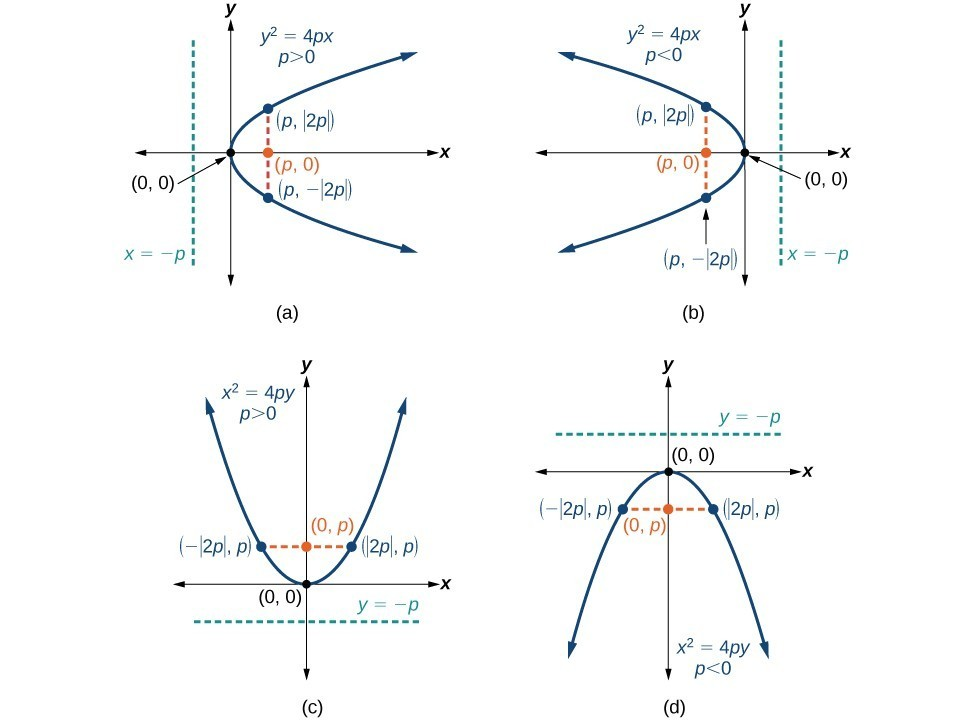(a) When $p>0$ and the axis of symmetry is the x-axis, the parabola opens right. (b) When $p<0$ and the axis of symmetry is the x-axis, the parabola opens left. (c) When $p<0$ and the axis of symmetry is the y-axis, the parabola opens up. (d) When $\text{ }p<0\text{ }$ and the axis of symmetry is the y-axis, the parabola opens down.

The key features of a parabola are its vertex, axis of symmetry, focus, directrix, and focal diameter. When given a standard equation for a parabola centered at the origin, we can easily identify the key features to graph the parabola.

A line is said to be tangent to a curve if it intersects the curve at exactly one point. If we sketch lines tangent to the parabola at the endpoints of the focal diameter, these lines intersect on the axis of symmetry.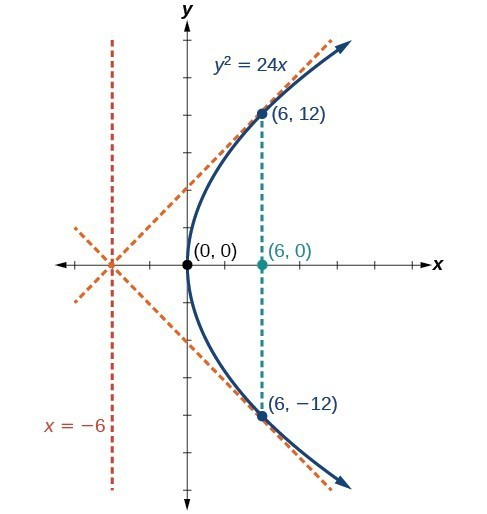### How To: Given a standard form equation for a parabola centered at (0, 0), sketch the graph.

• Determine which of the standard forms applies to the given equation: ${y}^{2}=4px$ or ${x}^{2}=4py$.
• Use the standard form identified in Step 1 to determine the axis of symmetry, focus, equation of the directrix, and endpoints of the focal diameter.
• If the equation is in the form ${y}^{2}=4px$, then
• the axis of symmetry is the x-axis, $y=0$
• set $4p$ equal to the coefficient of x in the given equation to solve for $p$. If $p>0$, the parabola opens right. If $p<0$, the parabola opens left.
• use $p$ to find the coordinates of the focus, $\left(p,0\right)$
• use $p$ to find the equation of the directrix, $x=-p$
• use $p$ to find the endpoints of the focal diameter, $\left(p,\pm 2p\right)$. Alternately, substitute $x=p$ into the original equation.
• If the equation is in the form ${x}^{2}=4py$, then
• the axis of symmetry is the y-axis, $x=0$
• set $4p$ equal to the coefficient of y in the given equation to solve for $p$. If $p>0$, the parabola opens up. If $p<0$, the parabola opens down.
• use $p$ to find the coordinates of the focus, $\left(0,p\right)$
• use $p$ to find equation of the directrix, $y=-p$
• use $p$ to find the endpoints of the focal diameter, $\left(\pm 2p,p\right)$
• Plot the focus, directrix, and focal diameter, and draw a smooth curve to form the parabola.

### Example: Graphing a Parabola with Vertex (0, 0) and the x-axis as the Axis of Symmetry

Graph ${y}^{2}=24x$. Identify and label the focus, directrix, and endpoints of the focal diameter.

### Try It

Graph ${y}^{2}=-16x$. Identify and label the focus, directrix, and endpoints of the focal diameter.

### Example: Graphing a Parabola with Vertex (0, 0) and the y-axis as the Axis of Symmetry

Graph ${x}^{2}=-6y$. Identify and label the focus, directrix, and endpoints of the focal diameter.

### Try It

Graph ${x}^{2}=8y$. Identify and label the focus, directrix, and endpoints of the focal diameter.

## Writing Equations of Parabolas in Standard Form

In the previous examples we used the standard form equation of a parabola to calculate the locations of its key features. We can also use the calculations in reverse to write an equation for a parabola when given its key features.

### How To: Given its focus and directrix, write the equation for a parabola in standard form.

• Determine whether the axis of symmetry is the x– or y-axis.
• If the given coordinates of the focus have the form $\left(p,0\right)$, then the axis of symmetry is the x-axis. Use the standard form ${y}^{2}=4px$.
• If the given coordinates of the focus have the form $\left(0,p\right)$, then the axis of symmetry is the y-axis. Use the standard form ${x}^{2}=4py$.
• Multiply $4p$.
• Substitute the value from Step 2 into the equation determined in Step 1.

### Example: Writing the Equation of a Parabola in Standard Form Given its Focus and Directrix

What is the equation for the parabola with focus $\left(-\frac{1}{2},0\right)$ and directrix $x=\frac{1}{2}?$

### Try It

What is the equation for the parabola with focus $\left(0,\frac{7}{2}\right)$ and directrix $y=-\frac{7}{2}$?

## Parabolas with Vertices Not at the Origin

Like other graphs we’ve worked with, the graph of a parabola can be translated. If a parabola is translated $h$ units horizontally and $k$ units vertically, the vertex will be $\left(h,k\right)$. This translation results in the standard form of the equation we saw previously with $x$ replaced by $\left(x-h\right)$ and $y$ replaced by $\left(y-k\right)$.

To graph parabolas with a vertex $\left(h,k\right)$ other than the origin, we use the standard form ${\left(y-k\right)}^{2}=4p\left(x-h\right)$ for parabolas that have an axis of symmetry parallel to the x-axis, and ${\left(x-h\right)}^{2}=4p\left(y-k\right)$ for parabolas that have an axis of symmetry parallel to the y-axis. These standard forms are given below, along with their general graphs and key features.

### A General Note: Standard Forms of Parabolas with Vertex (h, k)

The table summarizes the standard features of parabolas with a vertex at a point $\left(h,k\right)$.

 Axis of Symmetry Equation Focus Directrix Endpoints of focal diameter $y=k$ ${\left(y-k\right)}^{2}=4p\left(x-h\right)$ $\left(h+p,\text{ }k\right)$ $x=h-p$ $\left(h+p,\text{ }k\pm 2p\right)$ $x=h$ ${\left(x-h\right)}^{2}=4p\left(y-k\right)$ $\left(h,\text{ }k+p\right)$ $y=k-p$ $\left(h\pm 2p,\text{ }k+p\right)$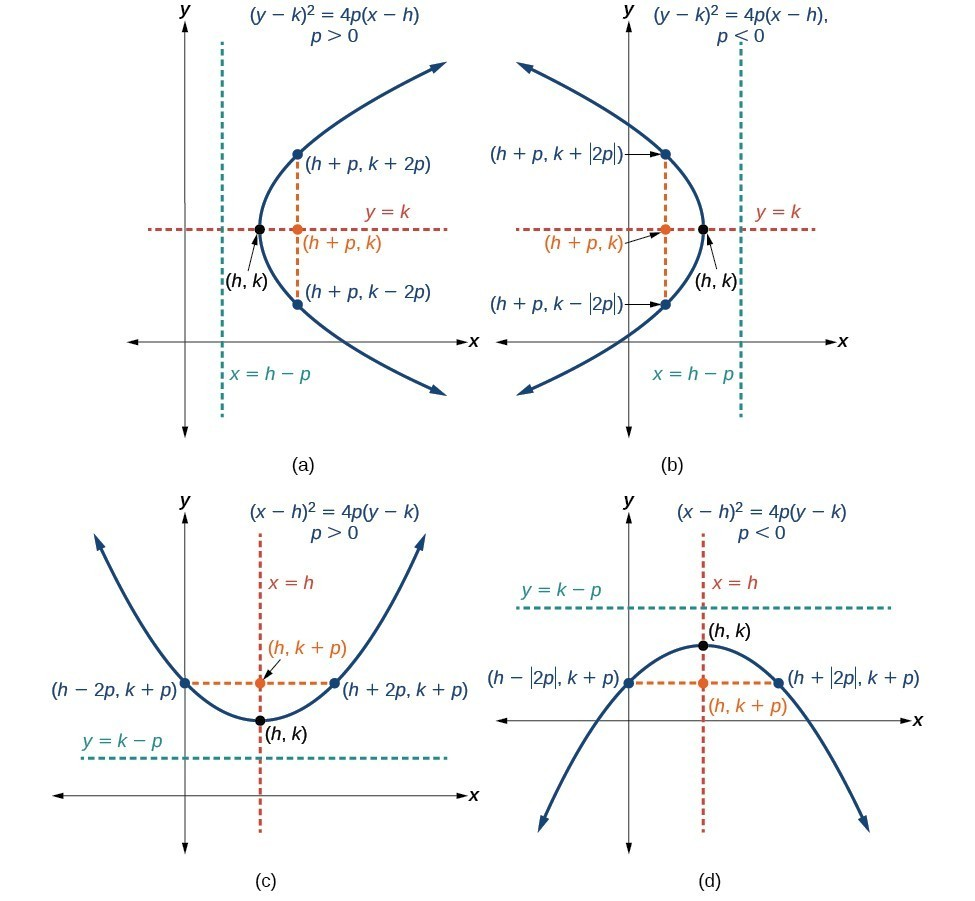(a) When $p>0$, the parabola opens right. (b) When $p<0$, the parabola opens left. (c) When $p>0$, the parabola opens up. (d) When $p<0$, the parabola opens down.

### How To: Given a standard form equation for a parabola centered at (h, k), sketch the graph.

1. Determine which of the standard forms applies to the given equation: ${\left(y-k\right)}^{2}=4p\left(x-h\right)$ or ${\left(x-h\right)}^{2}=4p\left(y-k\right)$.
2. Use the standard form identified in Step 1 to determine the vertex, axis of symmetry, focus, equation of the directrix, and endpoints of the focal diameter.
1. If the equation is in the form ${\left(y-k\right)}^{2}=4p\left(x-h\right)$, then:
• use the given equation to identify $h$ and $k$ for the vertex, $\left(h,k\right)$
• use the value of $k$ to determine the axis of symmetry, $y=k$
• set $4p$ equal to the coefficient of $\left(x-h\right)$ in the given equation to solve for $p$. If $p>0$, the parabola opens right. If $p<0$, the parabola opens left.
• use $h,k$, and $p$ to find the coordinates of the focus, $\left(h+p,\text{ }k\right)$
• use $h$ and $p$ to find the equation of the directrix, $x=h-p$
• use $h,k$, and $p$ to find the endpoints of the focal diameter, $\left(h+p,k\pm 2p\right)$
2. If the equation is in the form ${\left(x-h\right)}^{2}=4p\left(y-k\right)$, then:
• use the given equation to identify $h$ and $k$ for the vertex, $\left(h,k\right)$
• use the value of $h$ to determine the axis of symmetry, $x=h$
• set $4p$ equal to the coefficient of $\left(y-k\right)$ in the given equation to solve for $p$. If $p>0$, the parabola opens up. If $p<0$, the parabola opens down.
• use $h,k$, and $p$ to find the coordinates of the focus, $\left(h,\text{ }k+p\right)$
• use $k$ and $p$ to find the equation of the directrix, $y=k-p$
• use $h,k$, and $p$ to find the endpoints of the focal diameter, $\left(h\pm 2p,\text{ }k+p\right)$
3. Plot the vertex, axis of symmetry, focus, directrix, and focal diameter, and draw a smooth curve to form the parabola.

### Example: Graphing a Parabola with Vertex (h, k) and Axis of Symmetry Parallel to the x-axis

Graph ${\left(y - 1\right)}^{2}=-16\left(x+3\right)$. Identify and label the vertex, axis of symmetry, focus, directrix, and endpoints of the focal diameter.

### Try It

Graph ${\left(y+1\right)}^{2}=4\left(x - 8\right)$. Identify and label the vertex, axis of symmetry, focus, directrix, and endpoints of the focal diameter.

### Example: Graphing a Parabola from an Equation Given in General Form

Graph ${x}^{2}-8x - 28y - 208=0$. Identify and label the vertex, axis of symmetry, focus, directrix, and endpoints of the focal diameter.

### Try It

Graph ${\left(x+2\right)}^{2}=-20\left(y - 3\right)$. Identify and label the vertex, axis of symmetry, focus, directrix, and endpoints of the focal diameter.

## Solving Applied Problems Involving Parabolas

As we mentioned at the beginning of the section, parabolas are used to design many objects we use every day, such as telescopes, suspension bridges, microphones, and radar equipment. Parabolic mirrors, such as the one used to light the Olympic torch, have a very unique reflecting property. When rays of light parallel to the parabola’s axis of symmetry are directed toward any surface of the mirror, the light is reflected directly to the focus. This is why the Olympic torch is ignited when it is held at the focus of the parabolic mirror.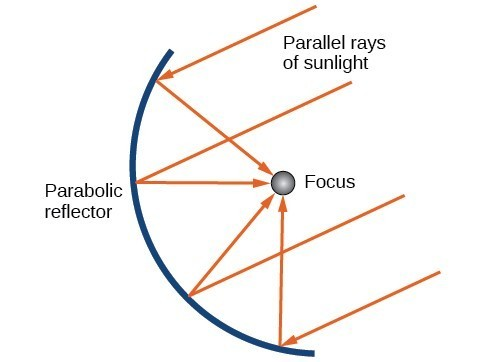Reflecting property of parabolas

Parabolic mirrors have the ability to focus the sun’s energy to a single point, raising the temperature hundreds of degrees in a matter of seconds. Thus parabolic mirrors are featured in many low-cost, energy efficient solar products, such as solar cookers, solar heaters, and even travel-sized fire starters.

### Example: Solving Applied Problems Involving Parabolas

A cross-section of a design for a travel-sized solar fire starter. The sun’s rays reflect off the parabolic mirror toward an object attached to the igniter. Because the igniter is located at the focus of the parabola, the reflected rays cause the object to burn in just seconds.

1. Find the equation of the parabola that models the fire starter. Assume that the vertex of the parabolic mirror is the origin of the coordinate plane.
2. Use the equation found in part (a) to find the depth of the fire starter.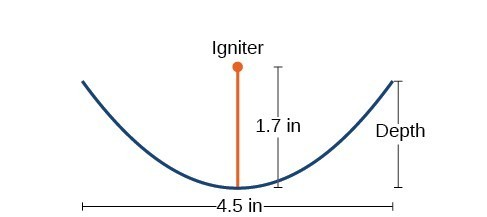Cross-section of a travel-sized solar fire starter

### Try It

Balcony-sized solar cookers have been designed for families living in India. The top of a dish has a diameter of 1600 mm. The sun’s rays reflect off the parabolic mirror toward the “cooker,” which is placed 320 mm from the base.

1. Find an equation that models a cross-section of the solar cooker. Assume that the vertex of the parabolic mirror is the origin of the coordinate plane, and that the parabola opens to the right (i.e., has the x-axis as its axis of symmetry).
2. Use the equation found in part (a) to find the depth of the cooker.

## Key Equations

 Parabola, vertex at origin, axis of symmetry on x-axis ${y}^{2}=4px$ Parabola, vertex at origin, axis of symmetry on y-axis ${x}^{2}=4py$ Parabola, vertex at $\left(h,k\right)$, axis of symmetry on x-axis ${\left(y-k\right)}^{2}=4p\left(x-h\right)$ Parabola, vertex at $\left(h,k\right)$, axis of symmetry on y-axis ${\left(x-h\right)}^{2}=4p\left(y-k\right)$

## Key Concepts

• A parabola is the set of all points $\left(x,y\right)$ in a plane that are the same distance from a fixed line, called the directrix, and a fixed point (the focus) not on the directrix.
• The standard form of a parabola with vertex $\left(0,0\right)$ and the x-axis as its axis of symmetry can be used to graph the parabola. If $p>0$, the parabola opens right. If $p<0$, the parabola opens left.
• The standard form of a parabola with vertex $\left(0,0\right)$ and the y-axis as its axis of symmetry can be used to graph the parabola. If $p>0$, the parabola opens up. If $p<0$, the parabola opens down.
• When given the focus and directrix of a parabola, we can write its equation in standard form.
• The standard form of a parabola with vertex $\left(h,k\right)$ and axis of symmetry parallel to the x-axis can be used to graph the parabola. If $p>0$, the parabola opens right. If $p<0$, the parabola opens left.
• The standard form of a parabola with vertex $\left(h,k\right)$ and axis of symmetry parallel to the y-axis can be used to graph the parabola. If $p>0$, the parabola opens up. If $p<0$, the parabola opens down.
• Real-world situations can be modeled using the standard equations of parabolas. For instance, given the diameter and focus of a cross-section of a parabolic reflector, we can find an equation that models its sides.

## Glossary

directrix a line perpendicular to the axis of symmetry of a parabola; a line such that the ratio of the distance between the points on the conic and the focus to the distance to the directrix is constant

focus (of a parabola) a fixed point in the interior of a parabola that lies on the axis of symmetry

focal diameter (latus rectum) the line segment that passes through the focus of a parabola parallel to the directrix, with endpoints on the parabola

parabola the set of all points $\left(x,y\right)$ in a plane that are the same distance from a fixed line, called the directrix, and a fixed point (the focus) not on the directrix